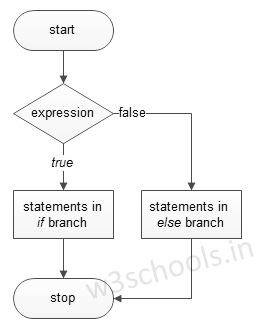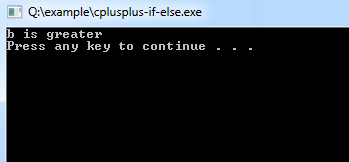## C++ Programming Tutorial Index

C++ `if-else` Statements control the flow of the program based on conditions. If the expression evaluates to `true`, it executes certain statements within the if block; Otherwise, it executes statements within the `else` code block. This tutorial will teach you how to use `if-else` Statements in C++.

The basic format of `if-else` Statement is:

Syntax:

``````if(test_expression)
{
}
else
{
}``````

Figure - Flowchart of `if-else` Statement:Example of a C++ program to demonstrate an `if-else` Statement:

Example:

``````#include <iostream>
using namespace std;

int main()
{
int a = 15, b = 20;

if (b > a) {
cout << "b is greater" << endl;
} else {
cout << "a is greater" << endl;
}
system("PAUSE");
}``````

Program Output:Example:

``````#include <iostream>
using namespace std;

int main()
{
char name;

cout << "Enter the name: "; cin >> name;
if (name == 'GG') {
}
else {
cout << "Incorrect PASSWORD, Try again.";
}
}
else {
cout << " Incorrect Login Details, Try again.";
}
}``````

Program Output:

```Enter the name: GG